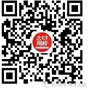#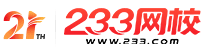计算机二级

•扫描二维码进入

• 题 库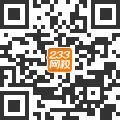扫描二维码进入

• 移动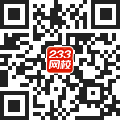233网校移动

• 移动APP扫描二维码下载APP

## 2019年3月计算机二级C语言考试选择题巩固练习019

1.若有定义语句：int a=3，b=2，c=1;，以下选项中错误的赋值表达式是(　　)。

A.a_(b=4)=3;

B.a=b=c+1;

C.a=(b=4)+c;

D.a=1+(b=c=4)：

2. 若有定义语句：intk1=10，k2=20;，执行表达式(k1=k1>k2)&&(k2=k2>k1)后，k1和k2的值分别为(　　)。

A.0和1

B.0和20

C.10和1

D.10和20

3.下列关系表达式中，结果为“假”的是

A.(3+4)>6

B.(3!=4)>2

C.3<=4||3

D.(3<4)==1

4.若有定义语句 int b=2; 则表达式(b<<2)/(3||b)的值是

A.4

B.8

C.0

D.2

5.下列程序段选择项，使i的运行结果为3的程序段是(　　)。

A.int i=0,j=0; (i=2,(j++)+i);

B.int i=1,j=0; j=i=((i=3)*2);

C. int i=0,j=1; (j==1)?(i=1):(i=3);

D.int i=1,j=1; i+=j+=1;

6.若变量均已正确定义并赋值，以下合法的C语言赋值语句是(　　)。

A.x=y==5;

B.x=n%2.5;

C.x+n=i;

D.x=5=4+1;

7.有以下定义：int a;long b;double x，y;则以下选项中正确的表达式是(　　)。

A.a%(int)(x-y)

B.a=x!=y;

C.(a*y)%b

D.y=x+y=x

8.设有定义：int x=3;，以下表达式中，值不为l2的是(　　)。

A.x*=x+1

B.x++，3*x

C.x*=(1+x)

D.2*x.x+=6

9.以下选项中非法的表达式是(　　)。

A.a+1=a+1

B.a=b==0

C.(Char.(100+100.

D.7<=X<60

10.若x=4，y=5，则x&y的结果是(　　)。

A.0

B.4

C.3

D.5

• 海量免费模拟试题，在线测试。

• 各科每年真题试卷，实战演练！

• 逐章逐节针对练习，专项突破。

• 每天10道最新试题，随机练习。

• QQ群号：806931213

扫描二维码加群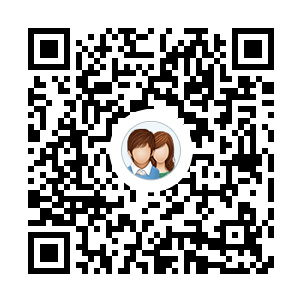• ##### 微信订阅号：kszx233

扫描二维码加群